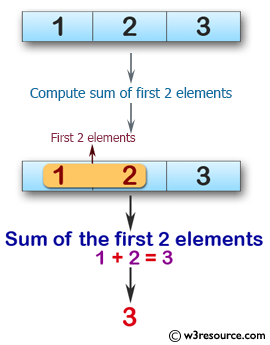﻿ Swift Array Programming Exercise: Compute the sum of the first 2 elements of a given array of integers. Return 0 if the lenght of the given array is 0 and return the first element value If the array length is less than 2 - w3resource# Swift Array Programming Exercises: Compute the sum of the first 2 elements of a given array of integers. Return 0 if the length of the given array is 0 and return the first element value If the array length is less than 2

## Swift Array Programming: Exercise-8 with Solution

Write a Swift program to compute the sum of the first 2 elements of a given array of integers. Return 0 if the length of the given array is 0 and return the first element value If the array length is less than 2.

Pictorial Presentation:Sample Solution:

Swift Code:

``````func array_sum2(_ a: [Int]) -> Int {
let size = a.count
if size>1
{
return a + a
}
else
{
return a
}
}

print(array_sum2([1, 2, 3]))
print(array_sum2([1, 1]))
print(array_sum2())
```
```

Sample Output:

```3
2
10
```

Swift Programming Code Editor:

Improve this sample solution and post your code through Disqus

What is the difficulty level of this exercise?

﻿